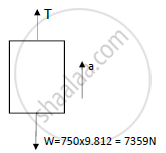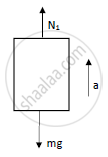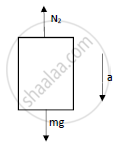Advertisement Remove all ads

# A 75kg Person Stands on a Weighing Scale in an Elevator. 3 Seconds After the Motion Starts from Rest, the Tension in the Hoisting Cable Was Found to Be 8300n. Find the Reading of the Scale, in - Engineering Mechanics

Answer in Brief

A 75kg person stands on a weighing scale in an elevator. 3 seconds after the motion starts from rest, the tension in the hoisting cable was found to be 8300N. Find the reading of the scale, in kg during this interval. Also find the velocity of the elevator at the end of this interval. The total mass of the elevator, including mass of the person and the weighing scale, is 750kg. If the elevator is now moving in the opposite direction, with same magnitude of acceleration, what will be the new reading of the scale?

Advertisement Remove all ads

#### Solution

t = 3 sec
u = 0 m/s a
T = 8300 N a
ΣF = ma
T – W = 750 x a
8300 – 7359 = 750 x a
a = 1.255 m/s2v = u + at
v = 0 + (1.255 x 3)
v = 3.765 m/sFor upward motion,

N1 – mg = ma
N1 = ma + mg
N1 = m(a+g)
N1 = 75(1.255+9.812)

N1 = 830.025 N
N1 = 84.59 kgFor downward motion, mg
N2 – mg = - ma
N2 = mg - ma
N2 = m(g-a) a
N2 = 75(9.812 – 1.255)
N2 = 641.775 N
N2 = 65.407 kg

In upward motion the reading on the weighing scale is 84.59 kg, final velocity at the end = 3.765 m/s and the reading on the weighing scale is 65.407 kg in the downward direction.

Concept: Velocity and Acceleration in Terms of Rectangular Co-ordinate System
Is there an error in this question or solution?
Advertisement Remove all ads

#### APPEARS IN

Advertisement Remove all ads
Advertisement Remove all ads
Share
Notifications

View all notifications

Forgot password?